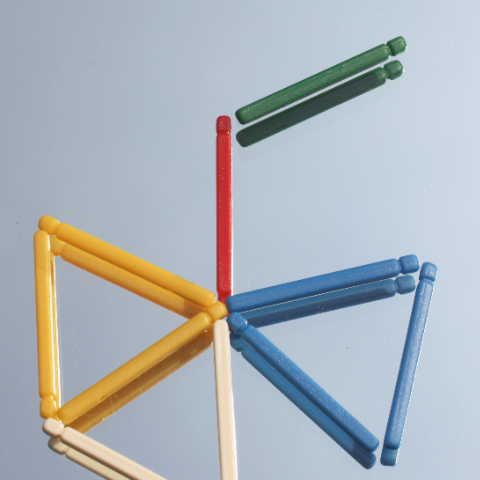# Philosophy of Mathematics Seminar (Monday - Week 6, MT22)Hilbert proposed, in his recently discovered 24th Problem, to investigate mathematical proofs; in 1918, he called for a “theory of the specifically mathematical proof”. Gentzen insisted in 1936 that “the objects of proof theory shall be the proofs carried out in mathematics proper” and viewed derivations in his natural deduction calculi as “formal images” of such proofs.  Clearly, these images were to represent significant structural features of the proofs from mathematical practice. The thorough formalization of mathematics encouraged Mac Lane to view formal derivations as starting-points for a theory of mathematical proofs.

These ideas for a theory of mathematical proofs have been reawakened by a confluence of investigations on natural reasoning (in the tradition of Gentzen and Prawitz), interactive verifications of theorems, and implementations of mechanisms that search for proofs. At this intersection of proof theory with interactive and automated proof construction, one finds a promising avenue for exploring the structure of mathematical proofs. I will detail steps down this avenue: the representation of proofs in formal logical frames is akin to the representation of physical phenomena in mathematical theories; an important dynamical aspect is captured through the articulation of bi-directional and strategically guided procedures for constructing (normal) proofs.

References:

Gentzen (1936) Die Widerspruchsfreiheit der reinen Zahlentheorie. Mathematische Annalen 112(1): 493-565.

Hilbert (1918) Axiomatisches Denken. Mathematische Annalen 78:405-415.

Mac Lane (1934) Abgekürzte Beweise im Logikkalkul. Dissertation, Georg-August-Universität, Göttingen.

Mac Lane (1935) A logical analysis of mathematical structure. The Monist 45(1):118-130.

Sieg & Byrnes (1998) Normal natural deduction proofs (in classical logic), Studia Logica 60: 67-109.

Sieg (2010) Searching for proofs (and uncovering capacities of the mathematical mind). Reprinted in: Sieg, Hilbert’s Programs and Beyond, OUP, 2013: 377-401.

Sieg & Walsh (2019) Natural formalization: Deriving the Cantor-Bernstein theorem in ZF. Review of Symbolic Logic, on-line 11/18/2019: 250-284.

Sieg & Derakhshan (2021), Human-centered automated proof search. Journal of Automated Reasoning 65: 1153-1190.

Thiele (2003) Hilbert's twenty-fourth problem. The American Mathematical Monthly 110(1):1-24.

Meeting will be in person. Those who wish to attend online, please write to Daniel Isaacson.

Philosophy of Mathematics Seminar Convenors: Daniel Isaacson and James Studd